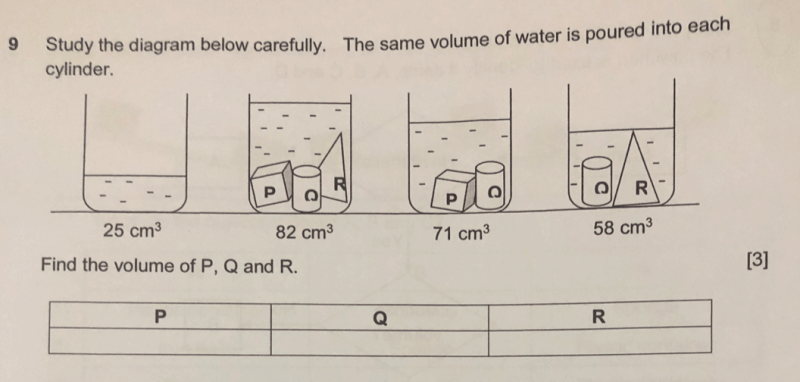# Question2 Answers

# Answer

Basically, to answer this question, you need to understand a few concepts:

1. The volume of the water in the cylinder is the same at 25cm3
2. Therefore you want to first simplify the problem by comparing something that has one less or one more item. In this case, that would be 2nd and 3rd or the 2nd and 4th. We shall go with the 2nd and 3rd option here. The only difference is that there is no R in the third. Therefore, R must be the difference

R: 82-71 = 11cm3

3.  Now that you know R is 11cm3, you can get Q by subtracting R and the volume of the water from the 4th cyclinder

Q: 58 – 25 – 11 = 22cm3

4. Finally, since you have these 2, you can find the final one by subtracting all the three values (including the water from point 1) from the 2nd jar

P: 82 – 25 – 11 – 22 = 24cm3

Alternatively, since you have both Q + R with water already in the 4th jar (which is 58cm3),
you can simply take the 2nd jar minus that value

P: 82 – 58 = 24cm3 (I prefer this method because less working means less careless mistakes)

5. I hope this helps explain the problem very clearly.
0 Replies 1 Like